# First Bianchi identity

(Redirected from Algebraic Bianchi identity)

## Statement

Let$\nabla$ be a torsion-free linear connection. The Riemann curvature tensor$R$ of$\nabla$ satisfies the following first Bianchi identity or algebraic Bianchi identity: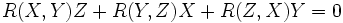$R(X,Y)Z + R(Y,Z)X + R(Z,X)Y = 0$

for any three vector fields$X,Y,Z$.

Notice that since this proof is applicable for any torsion-free linear connection, it in particular holds for the Levi-Civita connection arising from a Riemannian metric or pseudo-Riemannian metric.

## Proof

### Using repeated simplication and the Jacobi identity

Let us plug the definition of the Riemann curvature tensor: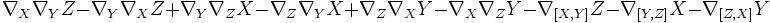$\nabla_X\nabla_YZ - \nabla_Y\nabla_XZ + \nabla_Y\nabla_ZX - \nabla_Z\nabla_YX + \nabla_Z\nabla_XY - \nabla_X\nabla_ZY - \nabla_{[X,Y]}Z - \nabla_{[Y,Z]}X - \nabla_{[Z,X]}Y$

This can be regrouped as: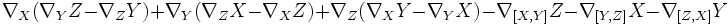$\nabla_X(\nabla_YZ - \nabla_ZY) + \nabla_Y(\nabla_ZX - \nabla_XZ) + \nabla_Z (\nabla_XY - \nabla_YX) - \nabla_{[X,Y]}Z - \nabla_{[Y,Z]}X - \nabla_{[Z,X]}Y$

Now, since$\nabla$ is torsion-free, we have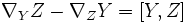$\nabla_YZ - \nabla_ZY = [Y,Z]$ and similar simplifications yield: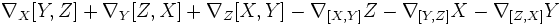$\nabla_X[Y,Z] + \nabla_Y[Z,X] + \nabla_Z[X,Y] - \nabla_{[X,Y]}Z - \nabla_{[Y,Z]}X - \nabla_{[Z,X]}Y$

again using the fact that$\nabla$ is torsion-free, this simplifies to:$[X,[Y,Z]] + [Y,[Z,X]] + [Z,[X,Y]]$

this becomes zero by the Jacobi identity.

### Using the differential Bianchi identity

Fill this in later